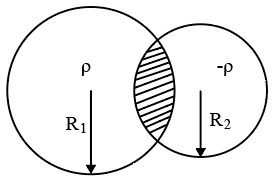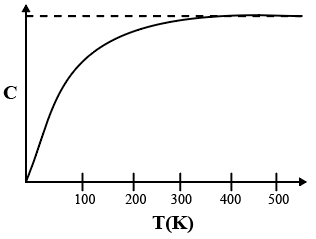Instructions

For the following questions answer them individually

Question 1

# Using the expression $$2d \sin \theta = \lambda$$, one calculates the values of d by measuring the corresponding angles $$\theta$$ in the range 0 to $$90^\circ$$. The wavelength $$\lambda$$ is exactly known and the error in $$\theta$$ is constant for all values of $$\theta$$. As $$\theta$$ increases from $$0^\circ$$,Question 2

# Two non-conducting spheres of radii $$R_1$$ and $$R_2$$ and carrying uniform volume charge densities $$\rho$$ and $$\rho$$ respectively, are placed such that they partially overlap, as shown in the figure. At all points in the overlapping region,Question 3

# The figure below shows the variation of specific heat capacity (C) of a solid as a function of temperature (T). The temperature is increased continuously from 0 to 500 K at a constant rate. Ignoring any volume change, the following statement(s)is (are) correct to a reasonable approximation.Question 4

# The radius of the orbit of an electron in a Hydrogen-like atom is 4.5 $$a_0$$, where $$a_0$$ is the bohr radius. Its orbital angular momentum is $$\frac{3h}{2 \pi}$$. It is given that h is Planck constant and R is Rydberg constant. The possible wavelength(s), when the atom de-excites, is (are)Question 5

# Two bodies, each of mass M, are kept fixed with a separation 2L. A particle of mass m is projected from the midpoint of the line joining their centres, perpendicular to the line. The gravitational constant is G. The correct statement(s) is (are)Question 6

# A particle of mass mis attached to one end of a mass-less spring of force constant k, lying on a frictionless horizontal plane. The other end of the spring is fixed. The particle starts moving horizontally from its equilibrium position at time t = 0 with an initial velocity $$u_0$$. When the speed of the particle is 0.5 $$u_0$$,it collides elastically with a rigid wail. After this collision,Question 7

# A steady current I flows along an infinitely long hollow cylindrical conductor of radius R. This cylinder is placed coaxially inside an infinite solenoid of radius 2R. The solenoid has n turns per unit length and carries a steady current I. Consider a point P at a distance r from the common axis. The correct statement(s) is (are)Question 8

# Two vehicles, each moving with speed u on the samehorizontal straight road, are approaching each other. Wind blows along the road with velocity w. One of these vehicles blows a whistle of frequency $$f_1$$. An observerin the other vehicle hears the frequency of the whistle to be $$f_2$$. The speed of soundin still air is V. The correct statement(s) is (are)Instructions

Following Questions of a paragraph has only one correct answer amongthe four choices(A), (B), (C) and (D).

A point charge Q is moving in a circular orbit of radius R in the x-y plane with an angular velocity $$\omega$$ This can be considered as equivalent to a loop carrying a steady current $$\frac{Q \omega}{2 \pi}$$. A uniform magnetic field along the positive z-axis is now switched on, which increases at a constant rate from 0 to B in one second. Assumethat the radius of the orbit remains constant. The application of the magnetic field induces an emf in the orbit. The induced
emf is defined as the work done by an induced electric field in moving a unit positive charge around a closed loop. It is knownthat, for an orbiting charge, the magnetic dipole momentis proportional to the angular momentum with a proportionality constant $$\gamma$$

Question 9

# The magnitude of the induced electric field in the orbit at any instant of time during the time interval of the magnetic field change isQuestion 10

# The change in the magnetic dipole moment associated with the orbit, at the end of the time interval of the magnetic field change, isOR